Thank you for visiting nature.com. You are using a browser version with limited support for CSS. To obtain the best experience, we recommend you use a more up to date browser (or turn off compatibility mode in Internet Explorer). In the meantime, to ensure continued support, we are displaying the site without styles and JavaScript.

# Wave instabilities in the presence of non vanishing background in nonlinear Schrödinger systems

## Abstract

We investigate wave collapse ruled by the generalized nonlinear Schrödinger (NLS) equation in 1+1 dimensions, for localized excitations with non-zero background, establishing through virial identities a new criterion for blow-up. When collapse is arrested, a semiclassical approach allows us to show that the system can favor the formation of dispersive shock waves. The general findings are illustrated with a model of interest to both classical and quantum physics (cubic-quintic NLS equation), demonstrating a radically novel scenario of instability, where solitons identify a marginal condition between blow-up and occurrence of shock waves, triggered by arbitrarily small mass perturbations of different sign.

## Introduction

Wave collapse, i.e. the occurrence of blow up in a finite time or propagation distance1,2,3, is a general phenomenon that appears in many contexts including self-focusing in optics4,5 (for an extensive review and a historical perspective, see ref. 6), plasma waves7, Bose-Einstein condensates8,9, hydrodynamics10 and organic systems11. Indeed several dispersive models with critical or supercritical nonlinearity (e.g. generalized KdV or KP equations12, modified KP13, Zhakarov equation14 and discrete or nonlocal version of the nonlinear Schrödinger equation15,16) exhibit formation of a point singularity from a large class of initial data. The critical case is by far the most intriguing one due to the sensitivity of the collapse to perturbations. In this context the most studied case, namely the focusing cubic nonlinear Schrödinger (NLS) equation, which is critical in two transverse dimensions, is still a formidable ground to understand the dynamics of collapse17,18,19,20 in spite of long standing investigations3,4,5,6. However, many physical classical and quantum systems need to be described in terms of generalized NLS (gNLS) equation which accounts for higher-order nonlinearities. Indeed such higher-order terms arise from different physical mechanisms in nonlinear optics (saturation of optical susceptibilities of standard material21,22,23, local field effects24, tailoring of nonlinearities in a cooled gas25), dynamics of superuids26, or quantum condensed systems where they are related to higher-order atom-atom interactions27,28,29. In gNLS systems critical collapse can occur also in one transverse dimension when quintic (or higher-order) nonlinearity is effective2,30,31. Though the blow-up problem is generally addressed for bright (i.e., zero background) solutions, gNLS systems support translationally invariant solitary wave solutions with non-zero background, yet of bright type32,33,34,35,36 (complement of well known dark soliton or bubble type solutions37,38), for which the problem of blow-up have been overlooked. Our aim in this paper is to establish a novel criterion for collapse valid for solutions of this type and study the dynamics across the threshold for blow-up. This allows us to reveal a new instability scenario where opposite behaviors, such as blowup or decay into a dispersive shock wave, can be controlled by means of an arbitrarily weak perturbation which controls the variation of the power (or mass) integral of a launched perturbed solitary wavepacket. We consider regimes for which the background itself is stable (otherwise modulation instability of the background becomes the main mechanism that affects the decay dynamics of the field).

The paper is organized as follows. In Section 2 we introduce the model and develop a sufficient criterion for collapse. In Section 3, we propose an approach to characterize non-collapsing solutions based on self-similar and semiclassical scaling arguments. The prediction of our analysis are confirmed by means of numerical simulations with reference to a specific model of physical interest in Section 4.

## The model and collapse criterion

We start from the following gNLS equation in dimensionless units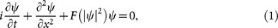with a general nonlinearity F(ρ) (henceforth ρ = |ψ|2, which has physical meaning of density or intensity in optics) and study the instability scenario of localized fields ψ characterized by nonvanishing boundary conditions:. Equation (1) is conveniently normalized by setting F0) = 0 at the background value ρ0. Invariants of motion originate from the translational symmetries along t, x and Gauge transformations, resulting in the renormalized functionals associated with energy H, momentum P and L2 norm M (henceforth referred to as ‘mass’, though it can physically represent number of particles or power in optics)39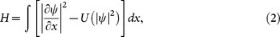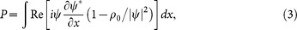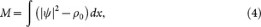where.

As demonstrated by Barashenkov38, traveling solitary solutions ψ(x, t) = ϕ(xvt) of the gNLS equation (1) on a finite background ρ0 ≠ 0 are unstable when the slope of the curve P = P(v) becomes negative (dP/dv < 0). Under the validity of such assumption, we begin our theoretical analysis by finding a sufficient criterion for collapse based on virial identities (see the review papers in Refs. 1,2,3, 6. We address the most common regime where, in the absence of an initial derivative of the virial (second-order momentum), the collapse of a bright disturbance with vanishing background is known to be essentially ruled by the energy (Hamiltonian) invariant of motion. Conversely, for a finite background we show that blow-up is driven by a suitable combination of renormalized energy and mass, thus allowing the existence of non collapsing solutions even for negative energy. We then employ a combination of virial identities and arguments based on self-similarity to study the leading order scaling of the dynamics in both collapsing and non collapsing regimes. In the latter case, a suitable rescaling in a semiclassical form and a diagonalization of the resulting equations of motion in terms of Riemann invariants, allow us to predict a completely novel scenario where collapse is arrested and the instability leads to the decay into dispersive shock waves, i.e. expanding fast wavetrains that are naturally emitted, owing to dispersion, to regularize steep gradients developing through the nonlinearities40,41,42,43,44,45,46,47,48,49,50,51. We verified our theoretical finding on a model of physical interest, namely the defocusing-focusing Cubic-Quintic Nonlinear Schrödinger Equation (CQNLS). We demonstrate a specific class of localized pulses on a pedestal, represented by antidark solitons, marking a marginal condition crossover between collapse and shock-wave generation, controlled via (even infinitesimal) mass perturbations. These findings, other than the fundamental interest related to collapse, suggest a new avenue for arresting blow-up and generating dispersive shocks from unstable solutions with nonzero background.

We begin our theoretical analysis by writing the Hamilton-Jacobi equations of motion for the evolution of a suitable renormalized virial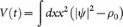: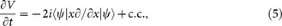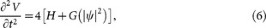where we set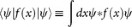and Eq. (6) is obtained by further deriving in time Eq. (5) and expressing such derivative in terms of the energy functional H and the suitably defined functional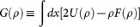, which depends on the nonlinear response of the system. In order to derive a sufficient criterion for collapse, we expand in series the functional G(ρ), obtaining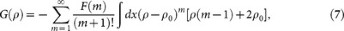with. Several different scenario can arise according to the signs of the terms in the expansion (7). An interesting case manifests when a low order defocusing nonlinearity —which supports localized solutions on a pedestal— competes with high order contributions of different sign, i.e., when F(1) < 0 and F(m) > 0 for m > 2. The following inequality then holds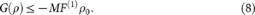Then, combining Eq. (6) and (8), we find the following differential inequality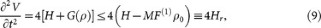which gives an upper bound to the dynamics of the virial V(t) in terms of rescaled Hamiltonian Hr, depending on the renormalized energy H and mass M. When Hr < 0, or equivalently: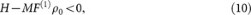the virial V(t) ~ Ct2 (C negative constant) tends to zero regardless of any initial condition V(0) and ∂V(0)/∂t. In order to understand the consequences of a vanishing virial on the dynamics of the field ψ, we employ a specific form of the Hölder inequality: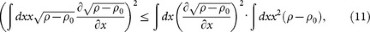and through an integration by parts of Eq. (11), derive the following upper limit for the virial evolution,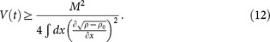According to Eq. (12), if the virial tends to zero, the gradient normdiverges in order to maintain an invariant mass M. This condition leads to the formation of a singularity in the field ρ − ρ0. Equation (10) can be therefore regarded as a sufficient condition for blow-up in the generalized NLS equation (1) with non vanishing boundary conditions. An important observation, stemming from Eq. (12), concerns the role of the background during the collapse. In particular the gradient norm, which drives the collapsing dynamics, does not depend on the background ρ0. The latter is therefore unaffected by the dynamics and the density evolves by respecting the boundary conditions ρ(±∞, t) = ρ0.

## Self-similar analysis and semiclassical argument

The differential inequality (9) can be also employed to calculate the leading order dynamics in both collapsing and non collapsing regimes. We start from the following self-similar form of the field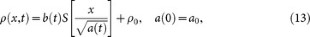which can be used to extract the scaling dynamics in the unstable regime3 and then employ the conservation of the mass M to determine the relationship between the peak value b and the width (variance) a of the field |ψ|2 − ρ0,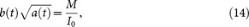being. By substituting Eqs. (13) and (14) into Eq. (9), we obtainhaving introduced the constantrepresenting the second-order moment of inertia of S(x) with respect to x = 0. By integrating twice Eq. (15) in time, we straightforwardly obtain the following lower bound for the evolution of the peak b of |ψ|2 − ρ0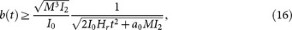which states that at leading order the field power b(t) scales asfor a non collapsing solution possessing Hr > 0. This scaling has important consequences in the development of shock wave instabilities when the input self-similar parameter is small, i.e., forwith. In this case, we can rescale Eq. (1) withand, thus obtaining a semiclassical form of the NLS equation with a general nonlinearity: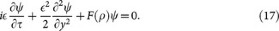Equation (17) can be casted into a hydrodynamic (or so-called dispersionless) form by applying the Madelung trans-formationand taking the leading-order terms in, which yields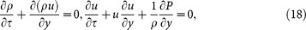which is physically equivalent to the system (also known as P-system) that rules the evolution of a compressible gas flow with density ρ, velocity u and pressure given through the equation of state P = P(ρ). In our case such equation of state is explicitly given in terms of nonlinearity and density as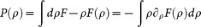, which in turn defines the sound velocity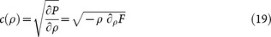Equivalently Eqs. (18) can be cast in the form of a system of quasi-linear equations ∂τq + Ayq = 0, with q ≡ (ρ, u)T and 2 × 2 matrix A = (u ρ;−∂ρF u), which is diagonalizable in the form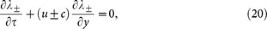by introducing the Riemann invariantsand the eigenvelocities of the system v± = u ± c, which correspond to the eigenvalues of the matrix A. By expanding in Taylor series the nonlinearity F(ρ) around ρ0, we obtain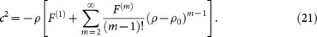When Eq. (10) is not fulfilled and a collapse is not observed in the dynamics, the field density decreases rapidly (as shown above by the self-similarity argument) and higher-order terms in the sum in Eq. (21) scale as, m ≥ 2, thus becoming negligible. As a consequence the velocity c is determined by the lowest power of ρ in the leading order term. For leading-order cubic nonlinearities where, with χ = ±1 is the sign of the nonlinearity, we have F(1) = χ and Eq. (21) yields. In this case, whenever χ = −1 (leading-order cubic nonlinearity of defocusing or repulsive type), the corresponding Riemann velocitiesare real and coincides (at leading order), with those pertaining to the integrable (cubic) defocusing NLS system41. As a consequence we expect the system to undergo wave-breaking through the development of a gradient catastrophe (formation of vertical front in finite time) from smooth input conditions launched in the system. In the former case the consequent regularization of the dynamics, owing to the dispersive terms neglected in Eqs. (17), occurs in term of a dispersive shock40,41,43,44,45,46,47,48,49,50,51. Conversely, such argument looses validity in the case χ = 1 (focusing cubic nonlinearity), wherewould imply a catastrophe of the elliptic umbilic type (characteristic of systems with dispersionless limit of the elliptic type with complex conjugate eigenvelocities v±), where ρ − ρ0 increases in time. In such a case higher-order terms in Eq. (21) become important and no conclusive statements on the type of dynamics can be made from such an approach.

In the following section we show, with reference to a specific system, how an arbitrarily small mass perturbation of a solitary waves with non-zero background can lead, depending on its sign, to completely different different decay scenarios implying either collapse or dispersive shock formation.

## Application to cubic-quintic nonlinearity

In order to illustrate our theoretical findings on a realistic system of physical interest, we consider the CQNLS equation with competing nonlinearities. The CQNLS is a general model for superfluidity26, nonlinear optics of saturable nonlinearities or cascading effects24,37 and systems of quantum condensed gases with elastic two- and three-body interactions27,28. The regime of interest here is a leading-order (cubic) nonlinearity of the defocusing type saturated by a focusing quintic nonlinearity, which is described by the nonlinear function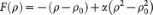, where α is a dimensionless parameter describing the strength of the quintic term (responsible for the collapse) over the defocusing cubic nonlinearity. We consider the following input field ρ(x, t = 0):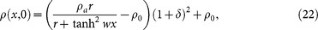where the small parameter δ is introduced to measures a mass perturbation of a still (zero velocity) anti-dark soliton ρsol(x) = ρar/(r + tanh2wx) [see profile in figure 1(a)], which is an exact solitary solution of the CQNLS provided r = ρ0/(ρa − ρ0),, ρa = (3 − 4αρ0)/2α35. Zero velocity anti-dark solitons of CQNLS are always unstable in the spirit of Ref. 38 while their background is stable35, thereby being ideal candidate for our theoretical investigation. We begin our analysis by calculating the correspondent reduced energy Hr, shown in figure 1(b). As seen, any positive mass perturbation δ > 0 leads to Hr < 0 and, by virtue of Eq. (10), to collapse. In order to numerically ascertain this result, we perform a direct integration of the CQNLS via a fourth order finite difference scheme. In our simulations, we launch Eq. (22) at t = 0 and monitor its dynamical evolution in the system for a fixed combination of α, ρ and a varying δ, which defines the mass perturbation of the CQNLS soliton. Our integration scheme is developed within an adaptive fourth order Runge-Kutta method52, which automatically adjusts the time resolution in order to maintain good accuracy on the results. The relative error tolerance in the simulation results is 10−9. The spatial resolution of the grid was dx = 8 · 10−3. During each time-step, we also monitored the conserved quantities (2), checking that their value remained practically constant. Figure 2 summarizes our results for instance for δ = 0.1, α = 0.1 and a background ρ0 = 1 (similar dynamics is obtained for other choices of values). In perfect agreement with our theory, a positive mass variation leads to a catastrophic collapse of the wave function [see figure 2(a–c)], which is attained at a relatively short distance, as shown in figure 2(c). We also observe, in agreement with Eq. (12), that the background intensity ρ0 does not change during the collapsing dynamics, remaining stable as also predicted by the linear stability analysis against the growth of periodic modulations35.

When the initial soliton mass is conversely decreased, for δ < 0, we have Hr > 0 [see figure 1(b)]. In the limit of a perturbative quintic nonlinearity α < 1, from Eq. (14) we obtain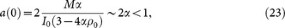which leads to a semiclassical behavior described by Eqs. (17) – (21) with. This predicts the generation of dispersive shock waves when δ < 0. Figure 3 reports numerical simulations for the case of a negative mass perturbation with δ = −0.01 and the same initial parameters (α = 0.1, ρ0 = 1) as in figure 2. In complete agreement with Eq. (16), the intensity amplitude falls off as[see figure 3(b)]. This process leads to wave breaking occurring through two symmetric gradient catastrophes (formation of symmetric steep fronts), followed by dispersive shock wave generation, as displayed in figure 3(c). It is worthwhile observing that for δ = −0.01 the energy H of the field ψ is still negative, as indicated by figure 1(b). At variance with the case of bright localized waveform on a zero background, which under the condition H < 0, are always collapsing in NLS systems3, our analysis demonstrates that a suitable background intensity can arrest the blow-up, leading to a completely different instability scenario where dispersive shocks are generated.

## Conclusions

In conclusion, we investigated wave instability in NLS systems in the presence of a non vanishing background. Through virial identities and semiclassical analysis, we have demonstrated a sufficient criterion for collapse and unveiled a novel instability dynamics characterized by the emission of dispersive shocks. Theoretical findings are verified against numerical simulations on the CQNLS equation, where we unveiled a set of solutions that exhibit a marginal crossover between the two aforementioned regimes. Besides the large interest of the physical community in wave collapse, these results show that a non vanishing background acts an affective mechanism for arresting collapse, leading to a new scenario characterized by the emission of shock waves.

## References

1. Kuznetsov, E. A., Rubenchik, A. & Zakharov, V. Soliton stability in plasmas and hydrodynamics. Phys. Rep. 142, 103–165 (1986).

2. Rasmussen, J. J. & Rypdal, K. Blow-up in nonlinear schroedinger equations-i a general review. Physica Scripta 33, 481–497 (1986).

3. Bergé, L. Wave collapse in physics: principles and applications to light and plasma waves. Phys. Rep. 303, 259 (1998).

4. Kelley, P. L. Self focusing of optical beams. Phys. Rev. Lett. 15, 1005 (1965).

5. Gaeta, A. L. Catastrophic collapse of ultrashort pulses. Phys. Rev. Lett. 84, 3582–3585 (2000).

6. Boyd, R. W., Lukishova, S. & Shen, Y. R. Self focusing: past and present (Springer, Topics in Applied Physics vol. 114, New York, 2009).

7. Wong, A. Y. & Cheung, P. Y. Three-dimensional self-collapse of langmuir waves. Phys. Rev. Lett. 52, 1222–1225 (1984).

8. Gerton, J. M., Strekalov, M., Prodan, I. & Hulet, R. G. Direct observation of growth and collapse of a bose-einstein condensate with attractive potential. Nature 408, 692–695 (2000).

9. Lahaye, T. et al. d-wave collapse and explosion of a dipolar bose-einstein condensate. Phys. Rev. Lett. 101, 080401 (2008).

10. Zeff, B. W., Kleber, B., Fineberg, J. & Lathrop, D. P. Singularity dynamics in curvature collapse and jet eruption on a fluid surface. Nature 403, 401–404 (2000).

11. Bang, O., Christiansen, P. L., If, F., Rasmussen, K. O. & Gaididei, Y. B. Temperature effects in a nonlinear model of monolayer scheibe aggregates. Phys. Rev. E 49, 4627–4636 (1994).

12. Wang, X., Ablowitz, M. & Segur, H. Wave collapse and instability of solitary waves of a generalized kadomtsev-petviashvili equation. Physica D 78, 241–265 (1994).

13. Whalen, P., Moloney, J. V., Newell, A. C., Newell, K. & Kolesik, M. Optical shock and blow-up of ultrashort pulses in transparent media. Phys. Rev. A 86, 033806 (2012).

14. Merle, F. Lower bounds for the blowup rate of solutions of the zakharov equation in dimension two. Communications on Pure and Applied Mathematics 49, 765–794 (1996).

15. Bang, O., Rasmussen, J. J. & Christiansen, P. L. Subcritical localization in the discrete nonlinear schrodinger equation with arbitrary power nonlinearity. Nonlinearity 7, 205–218 (1994).

16. Bang, O., Krolikowski, W., Wyller, J. & Rasmussen, J. J. Collapse arrest and soliton stabilization in nonlocal nonlinear media. Phys. Rev. E 66, 046619–(5) (2002).

17. Skupin, S., Saffman, M. & Królikowski, W. Nonlocal stabilization of nonlinear beams in a self-focusing atomic vapor. Phys. Rev. Lett. 98, 263902 (2007).

18. Linzon, Y., Rutkowska, K. A., Malomed, B. A. & Morandotti, R. Magneto-optical control of light collapse in bulk kerr media. Phys. Rev. Lett. 103, 053902 (2009).

19. Desyatnikov, A. S., Buccoliero, D., Dennis, M. R. & Kivshar, Y. S. Suppression of collapse for spiraling elliptic solitons. Phys. Rev. Lett. 104, 053902 (2010).

20. Shim, B., Schrauth, S. E., Gaeta, A. L., Klein, M. & Fibich, G. Loss of phase of collapsing beams. Phys. Rev. Lett. 108, 043902 (2012).

21. Chen, Y.-F. et al. Measurement of fifth- and seventh-order nonlinearities of glasses. J. Opt. Soc. Am. B 23, 347–352 (2005).

22. Béjot, P. et al. Higher-order kerr terms allow ionization-free filamentation in gases. Phys. Rev. Lett. 104, 103903 (2010).

23. Béjot, P. et al. Transition from plasma-driven to kerr-driven laser filamentation. Phys. Rev. Lett. 106, 243902 (2011).

24. Dolgaleva, K., Shin, H. & Boyd, R. W. Observation of a microscopic cascaded contribution to the fifth-order nonlinear susceptibility. Phys. Rev. Lett. 103, 113902 (2009).

25. Greenberg, J. A. & Gauthier, D. J. Higher-order optical nonlinearity at low light levels. Eur. Phys. Lett. 98, 24001 (2012).

26. Josserand, C. Cavitation induced by explosion in an ideal fluid model. Phys. Rev. E 60, 482–491 (1999).

27. Abdullaev, F. K., Gammal, A., Tomio, L. & Frederico, T. Stability of trapped bose-einstein condensates. Phys. Rev. A 63, 043604 (2001).

28. Pieri, P. & Strinati, G. C. Derivation of the gross-pitaevskii equation for condensed bosons from the bogoliuv̌de gennes equations for superfluid fermions. Phys. Rev. Lett. 91, 030401 (2003).

29. Kuznetsov, E. & Turitsyn, S. Instability and collapse of solitons in media with a defocusing nonlinearity. Sov. Phys. JETP 67, 1583–1588 (1988).

30. Glassey, R. T. On the blowing up of solutions to the cauchy problem for nonlinear schrödinger equations. J. Math. Phys. 18, 1794 (1977).

31. Chung, Y. & Lushnikov, P. M. Strong collapse turbulence in a quintic nonlinear schrödinger equation. Phys. Rev. E 84, 036602 (2011).

32. Kivshar, Y. S. & Afanasjev, V. V. Dark optical solitons with reverse-sign amplitude. Phys. Rev. A 44, R1446–R1449 (1991).

33. Frantzeskakis, D., Hizanidis, K., Malomed, B. & Polymilis, C. Stable anti-dark light bullets supported by the third-order dispersion. Physics Letters A 248, 203–207 (1998).

34. Coskun, T. H. et al. Bright spatial solitons on a partially incoherent background. Phys. Rev. Lett. 84, 2374–2377 (2000).

35. Crosta, M., Fratalocchi, A. & Trillo, S. Bistability and instability of dark-antidark solitons in the cubic-quintic nonlinear schrödinger equation. Phys. Rev. A 84, 063809 (2011).

36. Zakharov, V. E. & Gelash, A. A. Nonlinear stage of modulation instability. Phys. Rev. Lett. 111, 054101 (2013).

37. Kivshar, Y. S. & Luther-Davies, B. Dark optical solitons: physics and applications. Phys. Rep. 298, 81–197 (1998).

38. Barashenkov, I. V. Stability criterion for dark solitons. Phys. Rev. Lett. 77, 1193–1197 (1996).

39. Kivshar, Y. S. & Agrawal, G. P. Optical Solitons: from fibers to photonic crystals (Academic Press, San Diego, 2003).

40. Gurevich, A. V. & Pitaevskii, L. P. Nonstationary structure of a collisionless shock wave. Sov. Phys. JETP 38, 291 (1973).

41. Kamchatnov, A. M. Nonlinear Periodic Waves and Their Modulations (World Scientific, Singapore, 2000).

42. Kamchatnov, A. M., Gammal, A. & Kraenkel, R. A. Dissipationless shock waves in bose-einstein condensates with repulsive interaction between atoms. Phys. Rev. A 69, 063605 (2004).

43. El, G. A. Resolution of a shock in hyperbolic systems modified by weak dispersion. Chaos 15, 037103 (2005).

44. Fratalocchi, A., Conti, C., Ruocco, G. & Trillo, S. Free-energy transition in a gas of noninteracting nonlinear wave particles. Phys. Rev. Lett. 101, 044101 (2008).

45. Dutton, Z., Budde, M., Slowe, C. & Hau, L. V. Observation of quantum shock waves created with ultra- compressed slow light pulses in a bose-einstein condensate. Science 27, 293 (2001).

46. Hoefer, M. A. et al. Dispersive and classical shock waves in Bose-Einstein condensates and gas dynamics. Phys. Rev. A 74, 023623 (2006).

47. Meppelink, R. et al. Observation of shock waves in a large bose-einstein condensate. Phys. Rev. A 80, 043606 (2009).

48. Rothenberg, J. E. & Grischkowsky, D. Observation of the formation of an optical intensity shock and wave breaking in the nonlinear propagation of pulses in optical fibers. Phys. Rev. Lett. 62, 531–534 (1989).

49. Wan, W. J., Jia, S. & Fleischer, J. Dispersive superfluid-like shock waves in nonlinear optics. Nature Physics 3, 46 (2007).

50. Ghofraniha, N., Conti, C., Ruocco, G. & Trillo, S. Shocks in nonlocal media. Phys. Rev. Lett. 99, 043903 (2007).

51. Conti, C., Fratalocchi, A., Peccianti, M., Ruocco, G. & Trillo, S. Observation of a gradient catastrophe generating solitons. Phys. Rev. Lett. 102, 083902 (2009).

52. Shampine, L. F. (ed.) Numerical Solution of Ordinary Differential Equations (Chapman & Hall, New York, 1994).

## Acknowledgements

We acknowledge funding from Italian Ministry of University and Research (MIUR, grant PRIN 2012BFNWZ2) and KAUST (Award No. CRG-1-2012-FRA-005).

## Author information

Authors

### Contributions

A.F. and S.T. conceived the work and developed the theoretical analysis. J.S.T.G. performed the numerical simulations. A.F. and S.T. reviewed and wrote the manuscript.

## Ethics declarations

### Competing interests

The authors declare no competing financial interests.

## Rights and permissions

Reprints and Permissions